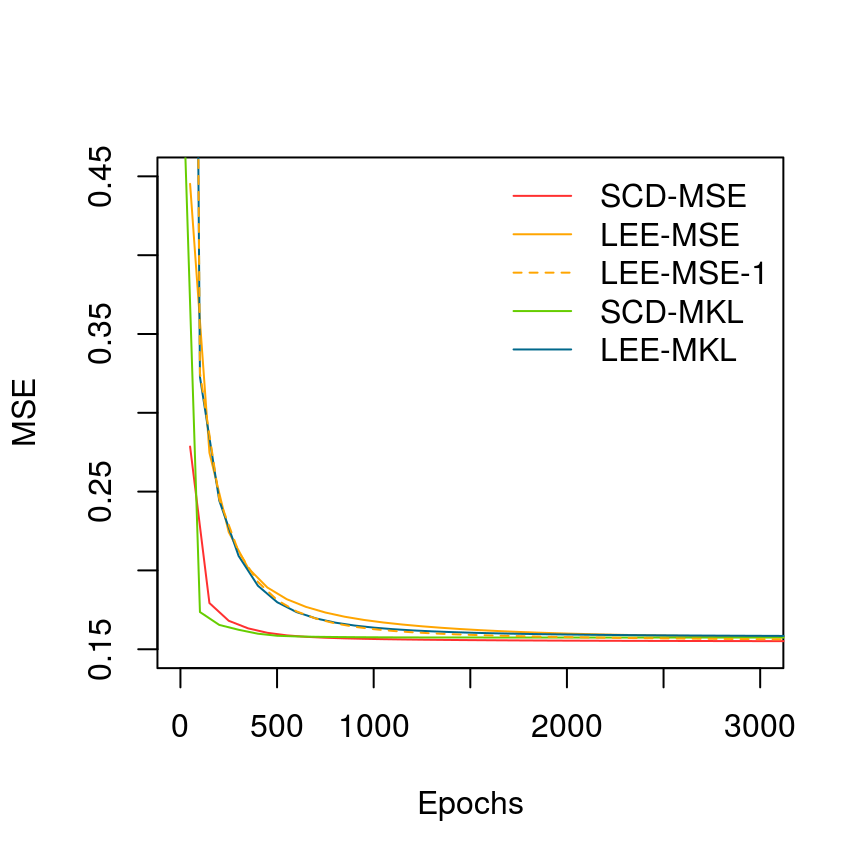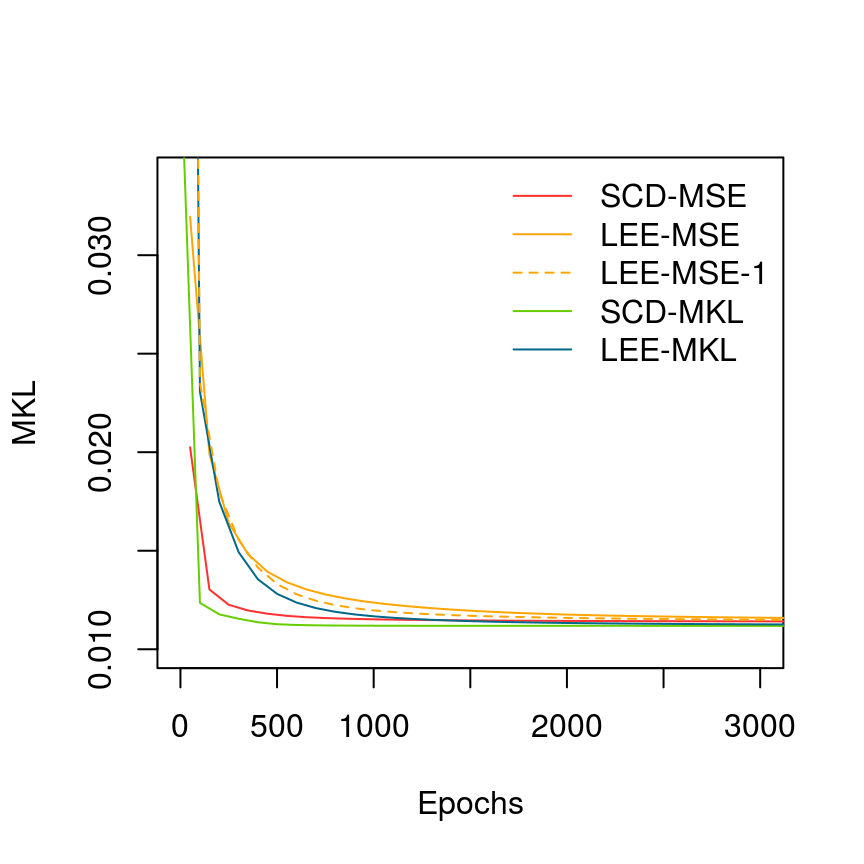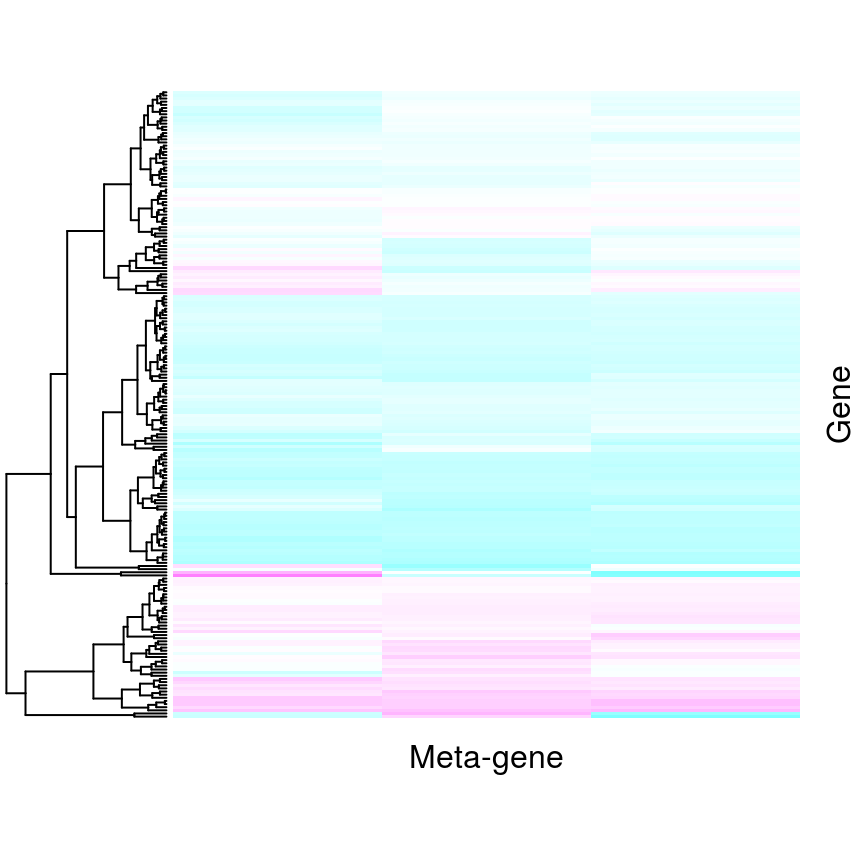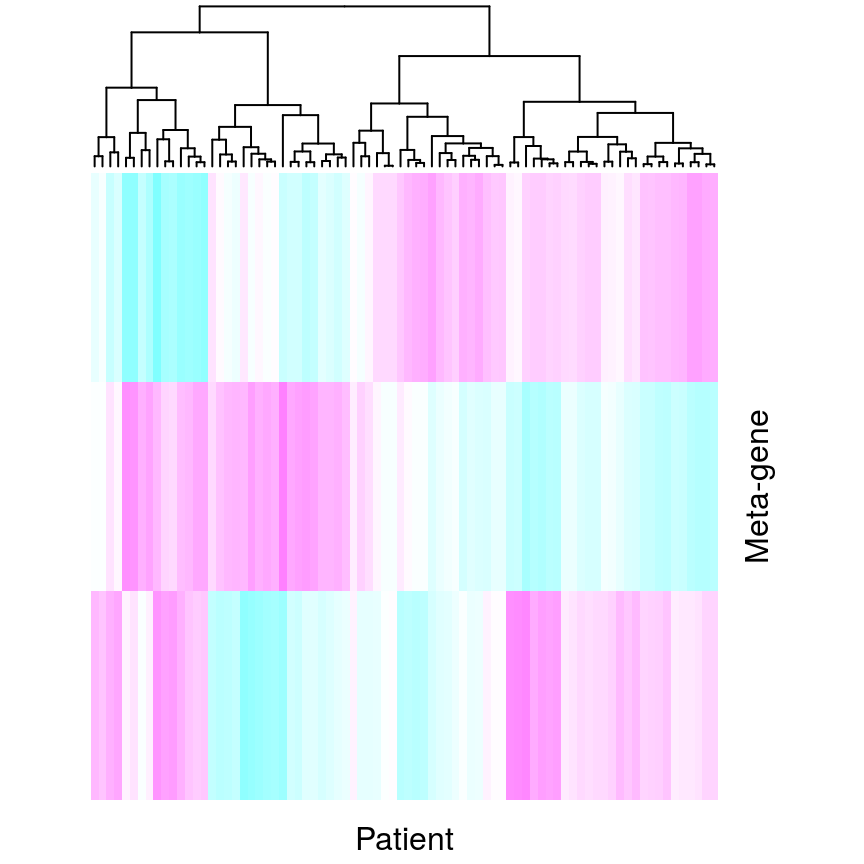# Background

Non-negative matrix factorization (NMF or NNMF) has been widely used as a general method for feature extraction on non-negative data. For example, meta-gene discovery from gene expression profiles in Kim and Park (2007), Brunet et al. (2004). There are currently a few algorithms for NMF decomposition, including the multiplicative algorithms proposed by Lee and Seung (1999), gradient decent and alternating non-negative least square (NNLS), which is getting popular recently, due to its speed to convergence.

# Software and implementation

The most popular R implantation of NMF is the NMF package (Gaujoux and Seoighe (2010)) which was first translated from a MATLAB package and later optimized via C++ for some algorithms. It implements various algorithms, such as the Lee’s multiplicative updates based on square error and Kullback-Leibler divergence distance, sparse alternating NNLS, etc. The sparse alternating NNLS (ALS) is supposed to be a very fast algorithm, but in practice, when number of rows increased to tens of thousands, this package gets very slow, probably due to its R implementation.

In NNLM, we adapt the ALS approach, but the NNLS problem is solved by a coordinate-wise algorithm proposed by Franc, Navara, and Hlavac (2005), in which each unknown variable can be solved sequentially and explicitly as simple quadratic optimization problems. Due to this efficient NNLS algorithm and the usage of Rcpp, our NMF is fast. Choice of different regularizations and many other unique features like integrating known profiles, designable factorization, missing value handling are also available.

# Description

This package includes two main functions, nnlm and nnmf.

## Sequential coordinate-wise descent (SCD)

nnlm solves the following non-negative least square (NNLS) $\min\limits_{\beta \ge 0} ||Y - X\beta||_F,$

using the following sequentially coordinate-wise algorithm (Franc, Navara, and Hlavac (2005)).

1. Let $$V = X^T X.$$
2. Initialize $$\beta^{(0)} = 0$$, $$\mu^{(0)} = -X^T Y.$$
3. Repeat until converge: for $$k = 1$$ to $$p$$, $\beta_k^{(t+1)} = \max\left(0,\,\beta_k^{(t)} - \dfrac{\mu_k^{(t)}}{v_{kk}}\right)$ $\mu^{(t+1)} = \mu^{(t)} + \left( \beta_k^{(t+1)} - \beta_k^{(t)}\right) V_{\cdot k}$ where $$V_{\cdot k}$$ is the $$k^{\mbox{th}}$$ coloum of $$V$$ and $$p$$ is the length of $$\beta$$.

Note that in this problem, $$Y$$ and $$X$$ are not necessary non-negative.

## Alternating NNLS with regularization

A typical non-negative matrix factorization problem can be expressed as $\min\limits_{W \ge 0, H \ge 0} \dfrac{1}{2}||A - WH||_F^2 + J_W(W) + J_H(H)$ where \begin{aligned} J_W(W) &= \alpha_1 J_1(W) + \alpha_2 J_2(W) + \alpha_3 J_3(W)\\ J_H(H) &= \beta_1 J_1(H^T) + \beta_2 J_2(H^T) + \beta_3 J_3(H^T) \end{aligned} and \begin{aligned} J_1(X) &:= \dfrac12 ||X||_F^2 = \dfrac12 \mbox{tr}(XX^T)\\% = \dfrac12 \sum\limits_{i, j} x_{ij}^2 \\ J_2(X) &:= \sum\limits_{i < j} (X_{\cdot i})^TX_{\cdot j} = \dfrac12 \mbox{tr}(X(E-I)X^T) \\ %= \dfrac12 \sum\limits_{i\neq j, k} x_{ki} x_{kj}\\ J_3(X) &:= \sum\limits_{i,j} |x_{ij}| = \mbox{tr}(XE) \\ J_4(X) &:= \dfrac12 \sum\limits_k ||X_{k\cdot}||_1^2 = \dfrac12 \mbox{tr}(XEX^T) %= \dfrac12 \sum\limits_{i, j, k} x_{ki} x_{kj}. \end{aligned}

In the above, $$A \in \mathbb{R}^{n\times m}, \, W \in \mathbb{R}^{n\times K}, \, H \in \mathbb{R}^{K\times m}$$, $$I$$ is an identity matrix, $$E$$ is a matrix of proper dimension with all entries equal to 1, $$w_{\cdot i}$$ and $$w_{i\cdot}$$ are the $$i^{\mbox{th}}$$ column and row respectively, $$h_{\cdot j}$$ is the j-th column of $$H$$. Obviously, $$J_4 = J_1 + J_2$$.

The above four types of regularizations can be used for different purposes. $$J_1$$ is a ridge penalty to control the magnitudes and smoothness. $$J_2(X)$$ is used to minimize correlations among columns, i.e., to maximize independence or the angle between $$X_{\cdot i}$$, $$X_{\cdot j}$$ (Zhang et al. (2008)). $$J_3$$ and $$J_4$$ (Kim and Park (2007)) is a LASSO like penalty, which controls both magnitude and sparsity. However, $$J_3(X)$$ tends control matrix-wise sparsity, but may also result in that some column of $$X$$ have all entries equal to $$0$$, while $$J_4(X)$$ forces sparsity in a column-wise manner, which should seldom give zero column.

The alternative lease square (ALS) algorithm solves $$W$$ and $$H$$ iteratively. Due to the non-negative constraint, the penalized NNLS is only slightly complicated. For example, When solving $$H$$ with $$W$$ fixed, i.e., minimizing for $$H$$ such that $$H\ge0$$, we have \begin{aligned} &\frac{1}{2}||A - WH||_F^2 + J_H(H) \\ =\, & \mbox{tr}\left\{\frac12 H^T \left[W^TW + \beta_1 I + \beta_2 (E-I)\right] H - H^T\left[W^TA- \beta_3 E\right]\right\} + const. \end{aligned}

Since $$E-I$$ is semi-negative definite, therefore, to ensure the uniqueness and the convergence of the algorithm, one has to ensure that $$W^TW + \beta_1 I + \beta_2 (E-I)$$ is positive definite. Indeed, we force a more stringent constraint that $$\beta_1 \ge \beta_2$$. Similarly, $$\alpha_1 \ge \alpha_2$$, since the ma. When the equality is reached, it is the $$J_4$$ constranint, i.e., row-wise LASSO for $$W$$ and column-wise LASSO for $$H$$.

The following algorithm is used to solved penalized NNLS.

1. Let $$V = W^T W + \beta_1 I + \beta_2 (E-I).$$
2. Initialization. Set $$H^{(0)} = 0$$, $$U^{(0)} = -W^TA + \beta_3 E.$$
3. Repeat until converge: for $$j = 1$$ to $$m$$, $$k = 1$$ to $$K$$ $h_{kj}^{(t+1)} = \max\left(0,\,h_{kj}^{(t)} - \dfrac{u_{kj}^{(t)}}{v_{kk}}\right)$ $U_{\cdot j}^{(t+1)} = U_{\cdot j}^{(t)} + \left( h_{kj}^{(t+1)} - h_{kj}^{(t)}\right) V_{\cdot k}$ where $$V_{\cdot k}$$ is the $$k^{\mbox{th}}$$ coloum of $$V$$.

The alternating NNLS algorithm fixes $$W$$ and solve for $$H$$ using NNLS, and then fixes $$H$$ and solve for $$W$$. This procedure is repeated until the change of $$A - W H$$ is small enough.

Instead of initializing $$H^{(0)} = 0$$ for every iteration, we use warm-start, i.e., make use of the previous iteration result, $H^{(0)} = H, \quad U^{(0)} = W^TWH - W^TA + \beta_3 E,$ where $$H$$ is the solution from the previous iteration.

## Sequential quadratic approximation for Kullback-Leibler divergence loss

A well known problem about square error loss is that it is not robust to skewed distribution (e.g., count data) or outliers. An alternative choice of loss function is the Kullback-Leibler divergence “distance” defined as $L(A, \hat A) = \mbox{KL}(A | \hat A) = \sum_{i,j} a_{ij} \log\dfrac{a_{ij}}{\hat a_{ij}} - a_{ij} + \hat a_{ij}.$ It can be proved that $$\mbox{KL}(A | \hat A) \ge 0$$ and equality is reached if and only if $$A = \hat A$$. In NMF, $$\hat A = W H$$.

One explanation of the the $$\mbox{KL}$$ divergence is to assume that $$a_{ij}$$ is some count drawn independently as $a_{ij} \,\sim\, \mbox{Poisson}(\lambda_{ij}).$ The correpondent log likelihood is $l(\Lambda) = \sum_{i, j} \left(a_{ij}\log\lambda_{ij} - \lambda_{ij}\right) + const.$ Maximizing this log likelihood with respect to $$\Lambda = \{\lambda_{ij}\}$$ is equivalent minimizing $$\mbox{KL}$$ with respect to $$\hat A$$. Assume that the observed $$a_{ij}$$ comes from a mixture of independent Poisson distributions with rates $$\lambda_{ij}^{(1)}, \dots, \lambda_{ij}^{(k)}$$, then $a_{ij} \,\sim\, \mbox{Poisson}\left(\lambda_{ij} = \sum_{l=1}^K\lambda_{ij}^{(l)}\right).$ If we force a structure $$\lambda_{ij}^{(l)} = w_{il} h_{lj}$$, we get NMF with $$\mbox{KL}$$ divergence loss.

With $$\mbox{KL}$$ divergence loss, a non-negative matrix factorization problem can be expressed as $\min\limits_{W \ge 0, H \ge 0} \mbox{KL}(A | W H) + J_W(W) + J_H(H).$

This problem can be solved using a similar alternating coordinate-wise algorithm, by approximating $$\mbox{KL}(A | W H)$$ with a quadratic function (Taylor expansion up to second order around its current value).

Assume $$W$$ is known and $$H$$ is to be solved. Let \begin{aligned} b :=& \dfrac{\partial \mbox{KL}}{\partial h_{kj}} \left(H^0\right) = \sum_{l}\left(w_{lk} - \frac{a_{lj}w_{lk}}{\sum_{q} w_{lq} h_{qj}^0}\right)\\ % + (\beta_1 - \beta_2) h_{kj}^0 + \beta_2 \sum_l h_{lj}^0 + \beta_3,\\ a :=& \dfrac{\partial^2\mbox{KL}}{\partial h_{kj}^2} \left(H^0\right) = \sum_{l} a_{lj} \left(\dfrac{w_{lk}}{\sum_{q} w_{iq} h^0_{qj}}\right)^2 % + \beta_1, \end{aligned} where $$H^0$$ is the current value of $$H$$ in the iteration procedure.

Therefore for $$h_{kj}$$, with all other entries fixed, $\mbox{KL}(A | W H) + J_H(H) \approx \frac12 a (h_{kj} - h_{kj}^0)^2 + b (h_{kj} - h_{kj}^0) + \frac12 \beta_1 h_{kj}^2 + \beta_2 h_{kj}\sum_{l\neq k}h^0_{lj} + \beta_3 h_{kj} + const,$ which can be minimized explicitly with constraint $$h_{kj} \ge 0$$, as $h_{kj} = \max\left(0, \, \dfrac{a h^0_{kj} - b - \beta_2 \sum_{l\neq k} h^0_{lj} - \beta_3}{a + \beta_1} \right).$

Obviously, this update can be parallelled in a column-wise manner.

Note that when an entry of $$\hat A$$ is 0, the $$\mbox{KL}$$ divergence is infinity. To avoid this, we add a very small number both to $$A$$ and $$\hat A$$.

Two multiplicative updating algorithms are proposed in Lee and Seung (1999) for square loss and KL divergence loss. We modify these algorithms to integrate all the above regularizations as the followings.

With square loss \begin{aligned} w_{ik} &= w_{ik} \dfrac{(AH^T)_{ik}}{\left(W\left[HH^T + \alpha_1 I + \alpha_2 (E-I)\right] + \alpha_3E \right)_{ik}},\\ h_{kj} &= h_{kj} \dfrac{(W^TA)_{kj}}{\left(\left[W^TW + \beta_1 I + \beta_2 (E-I)\right]H + \beta_3E \right)_{kj}}. \end{aligned}

With Kullback-Leibler divergence distance, \begin{aligned} w_{ik} &= w_{ik} \dfrac{\sum_{l=1} h_{kl}a_{il}/\sum_{q}w_{iq}h_{ql}} {\left(\sum_{l} h_{kl} + (\alpha_1-\alpha_2) w_{ik} + \alpha_2 \sum_{l} w_{il} + \alpha_3 \right)},\\ h_{kj} &= h_{kj} \dfrac{\sum_{l} w_{lk}a_{lj}/\sum_{q}w_{lq} h_{qj}} { \left( \sum_{l} w_{lk} + (\beta_1-\beta_2) h_{kj} + \beta_2 \sum_{l} h_{lj} + \beta_3 \right) }. \end{aligned}

When $$\alpha_i = 0, \beta_i = 0, i = 1, 2, 3$$, these are the original multiplicative algorithms in Lee and Seung (1999).

This multiplicative algorithm is straight forward to implemented, but it has a drawback that when $$W$$ or $$H$$ is initialized with a zero entry or positive, it remains $$0$$ or positive through iterations. Therefore, true sparsity can not be achieved generally, unless a hard-thresholding is forced, as many of entries should be small enough to be thresholded as 0.

## Complexity and convergence speed

One can easily see that both the sequential coordinate descent (SCD) and Lee’s multiplictive algorithms using MSE have complexity of $$\mathcal{O}\left(\left((m+n)k^2 N_i + 2nmK\right)N_o\right)$$, while their KL conterparts have complexity of $$\mathcal{O}\left(nmk^2N_iN_o\right)$$. Here $$N_i$$ is the number of inner iterations to solve the non-negative linear model and $$N_o$$ is the number of outer iteration to alternate $$W$$ and $$H$$. $$N_i x N_o$$ is the total number of epochs, i.e., swaps over $$W$$ and $$H$$ matrices. Obviously algorithm with MSE are faster then the KL based ones (by a factor of $$k$$) in terms of complexity, and can benefit from multiple inner iterations $$N_i$$ (reducing the expensive computation of $$W^TA$$ and $$AH^T$$) as typically $$k \ll m, n$$, which generally should reduce $$N_o$$. On the contrast, algorithm with KL has not benefit from $$N_i$$ due to the re-calculation of $$WH$$ on each inner iteration. Though the SCD and Lee’s algorithm are close in terms of complexity, one can stil expect that SCD will converge much faster in practice. This is because Lee’s multiplicative algorithm is indeed a gradient descent with a special step size (Lee and Seung (1999)) which is first order method, while the sequential coordinate-wise is a second order approach like the Newton-Raphson algorithm.

## Usage

One can install NNLM like

install.packages("NNLM");

# or get a dev-version from Github
library(devtools);
install_github('linxihui/NNLM')

### Interface

nnlm(x, y, alpha = rep(0,3), method = c('scd', 'lee'), loss = c('mse', 'mkl'), init = NULL,
mask = NULL, check.x = TRUE, max.iter = 10000L, rel.tol = 1e-12, n.threads = 1L)

nnmf(A, k = 1L, alpha = rep(0,3), beta = rep(0,3), method = c("scd", "lee"), loss = c("mse", "mkl"),
init = NULL, mask = NULL, W.norm = -1L, check.k = TRUE, max.iter = 500L, rel.tol = 1e-4,
n.threads = 1L, trace = 10L, verbose = 1L, show.warning = TRUE,
inner.max.iter = ifelse('mse' == loss, 50L, 1L), inner.rel.tol = 1e-09)
• x : design matrix.
• y : a vector or matrix of responses.
• A : matrix to decompose.
• k : rank of NMF.
• method: sequential coordinate descent ("scd") or Lee’s multiplicative algorithm ("lee").
• metric: loss function, mean square error or KL-divergence.
• init:
• nnlm: a non-negative matrix of initials.
• nnmf: a list of named initial matrices for $$W$$ and $$H$$. One can also supply known matrices $$W_0,\, H_0$$, and initialize their correspondent matrices $$H_1$$ and $$W_1$$.
• mask:
• nnlm: a logical matrix indicating if entries are fixed to 0 or initials if available.
• nnmf: a list of named mask matrices for $$W,\, H,\, H_1$$ (if init$W0 supplied), $$W_1$$ (if init$H0 supplied), which should have the same shapes as $$W,\, H,\, H_1$$ and $$W_1$$ if specified. If initial matrices not specified, masked entries are fixed to 0.
• alpha and beta: a vector of length equal to or less than 3, indicating $$\alpha_1, \alpha_2, \alpha_3$$ and $$\beta_1, \beta_2, \beta_3$$. If latter entries are missing, 0 is assumed.
• trace: an integer $$n$$ indicating how frequent the error should be checked, i.e., $$n$$-iterations.
• verbose: either 0/FALSE, 1/TRUE or 2, indicating no printing, progression bar or iteration details.
• n.threads: number of openMP threads.
• max.iter and rel.tol: number of outer alternating iterations and the relative tolerance.
• inner.max.iter and inner.rel.tol: number of inner iterations for solving NNLM and the relative tolerance. Default to 50 if loss == 'mse' and 1 if loss == 'mkl'.

## Compare different algorithms

library(NNLM);
set.seed(123);

k <- 15;
init <- list(W = matrix(runif(nrow(nsclc)*k), ncol = k),
H = matrix(runif(ncol(nsclc)*k), nrow = k));
# Set rel.tol = -1 to prevent from early stop, just for comparison purpose
scd.mse  <- nnmf(nsclc, k, init = init, max.iter = 100, rel.tol = -1, show.warning = FALSE);
lee.mse  <- nnmf(nsclc, k, init = init, max.iter = 100, rel.tol = -1, method = 'lee', show.warning = FALSE);
scd.mkl  <- nnmf(nsclc, k, init = init, max.iter = 5000, rel.tol = -1, loss = 'mkl', show.warning = FALSE);
lee.mkl  <- nnmf(nsclc, k, init = init, max.iter = 5000, rel.tol = -1, loss = 'mkl',
method = 'lee', show.warning = FALSE);
lee.mse1 <- nnmf(nsclc, k, init = init, max.iter = 5000, rel.tol = -1, method = 'lee',
inner.max.iter = 1, show.warning = FALSE);
plot(NULL, xlim = c(1, 3000), ylim = c(0.15, 0.45), xlab = 'Epochs', ylab = 'MSE');
lines(cumsum(scd.mse$average.epochs), scd.mse$mse, col = 'firebrick1');
lines(cumsum(lee.mse$average.epochs), lee.mse$mse, col = 'orange');
lines(cumsum(scd.mkl$average.epochs), scd.mkl$mse, col = 'chartreuse3');
lines(cumsum(lee.mkl$average.epochs), lee.mkl$mse, col = 'deepskyblue4');
lines(cumsum(lee.mse1$average.epochs), lee.mse1$mse, col = 'orange', lty = 2);
legend('topright', bty = 'n', lwd = 1, lty = c(1,1,2,1,1),
legend = c('SCD-MSE', 'LEE-MSE', 'LEE-MSE-1', 'SCD-MKL', 'LEE-MKL'),
col = c('firebrick1', 'orange', 'orange', 'chartreuse3', 'deepskyblue4'));

plot(NULL, xlim = c(1, 3000), ylim = c(0.01, 0.034), xlab = 'Epochs', ylab = 'MKL');
lines(cumsum(scd.mse$average.epochs), scd.mse$mkl, col = 'firebrick1');
lines(cumsum(lee.mse$average.epochs), lee.mse$mkl, col = 'orange');
lines(cumsum(scd.mkl$average.epochs), scd.mkl$mkl, col = 'chartreuse3');
lines(cumsum(lee.mkl$average.epochs), lee.mkl$mkl, col = 'deepskyblue4');
lines(cumsum(lee.mse1$average.epochs), lee.mse1$mkl, col = 'orange', lty = 2);
legend('topright', bty = 'n', lwd = 1, lty = c(1,1,2,1,1),
legend = c('SCD-MSE', 'LEE-MSE', 'LEE-MSE-1', 'SCD-MKL', 'LEE-MKL'),
col = c('firebrick1', 'orange', 'orange', 'chartreuse3', 'deepskyblue4'));

summary.nnmf <- function(x) {
if (x$n.iteration < 2) { rel.tol <- NA_real_; } else { err <- tail(x$target.loss, 2);
rel.tol <- diff(err)/mean(err);
}
return(c(
'MSE' = tail(x$mse, 1), 'MKL' = tail(x$mkl, 1), 'Target' = tail(x$target.loss, 1), 'Rel. tol.' = abs(rel.tol), 'Total epochs' = sum(x$average.epochs),
'# Interation' = x$n.iteration, x$run.time[1:3]));
};

library(knitr);
kable(
sapply(
X = list(
'SCD-MSE' = scd.mse,
'LEE-MSE' = lee.mse,
'LEE-MSE-1' = lee.mse1,
'SCD-MKL' = scd.mkl,
'LEE-MKL' = lee.mkl
),
FUN = function(x) {z <- summary(x); sapply(z, sprintf, fmt = '%.4g')}
),
align = rep('r', 5),
caption = 'Compare performance of different algorithms'
);
Compare performance of different algorithms
SCD-MSE LEE-MSE LEE-MSE-1 SCD-MKL LEE-MKL
MSE 0.155 0.1565 0.1557 0.1574 0.1579
MKL 0.01141 0.01149 0.01145 0.01119 0.01122
Target 0.07749 0.07825 0.07783 0.01119 0.01122
Rel. tol. 1.325e-05 0.0001381 0.000129 6.452e-08 9.739e-05
Total epochs 5000 5000 5000 5000 5000
# Interation 100 100 5000 5000 5000
user.self 1.522 1.868 7.924 36.45 33.55
sys.self 2.098 2.317 10.91 29.78 30.38
elapsed 0.925 1.058 4.841 26.86 24.55One can see from the above summary, the SCD and Lee’s algorithms have roughly the same run time for each epoch, i.e., updating $$W$$ and $$H$$ entries once. However, SCD generally converges much faster than Lee’s, i.e., achieving the same accuracy in less epochs/iterations and thus shorter time. Obviously, algorithms with mean KL loss are slower than those with MSE for each epoch, but reducing error a bit more in each epoch. Lee’s multiplicative algorith with MSE is faster when multiple epochs used in each outer alternating iteration (LEE-MSE vs LEE-MSE-1).

# Applications

## Pattern extraction

In Lee and Seung (1999), NMF is shown to be able to learn sparse basis images (column of $$W$$) that represent facial parts , like mouses, noses and eyes of different shapes. Each face (column of $$A$$) is a positive combinations of a few of these basis images. In topic discovery, NMF can learn to group documents into topics, where $$A$$ is a bag-of-word representation (count of a word in a document) of a batch of documents, with rows = vocabulary, and columns = documents. In NMF, the columns of $$W$$ (after normalization) represent topics (a topic is represented as the distribution over vocabulary), and a column of $$H$$ shows the topics a document covered.

In bioinformatics, NMF can be used to discover ‘meta-genes’ from expression profile, which are linear combinations of genes that may or may not related to some biology path ways. In this case, $$A$$ is usually arranged as rows = genes and columns = patients. The columns of $$W$$ can then be interpreted as meta-genes, and $$H$$ are said to be the expression profile of meta-genes (Brunet et al. (2004), Kim and Park (2007)). Alexandrov et al. (2013) used NMF to extract trinucleotide mutational signatures (basis) from next generation sequencing data (NGS) for human genes and discovered that each cancer type is combination of these basis.

library(NNLM);
set.seed(123);

data(nsclc, package = 'NNLM')
str(nsclc)
##  num [1:200, 1:100] 7.06 6.41 7.4 9.38 5.74 ...
##  - attr(*, "dimnames")=List of 2
##   ..$: chr [1:200] "PTK2B" "CTNS" "POLE" "NIPSNAP1" ... ## ..$ : chr [1:100] "P001" "P002" "P003" "P004" ...
decomp <- nnmf(nsclc[, 1:80], 3, rel.tol = 1e-5);
decomp
## Non-negative matrix factorization:
##    Algorithm: Sequential coordinate-wise descent
##         Loss: Mean squared error
##          MSE: 0.3481353
##          MKL: 0.02518784
##       Target: 0.1740676
##    Rel. tol.: 9.41e-06
## Total epochs: 2551
## # Interation: 81
## Running time:
##    user  system elapsed
##   0.078   0.002   0.081
heatmap(decomp$W, Colv = NA, xlab = 'Meta-gene', ylab = 'Gene', margins = c(2,2), labRow = '', labCol = '', scale = 'column', col = cm.colors(100)); heatmap(decomp$H, Rowv = NA, ylab = 'Meta-gene', xlab = 'Patient', margins = c(2,2),
labRow = '', labCol = '', scale = 'row', col = cm.colors(100));We can also use the derived meta-genes and find their expressions in new patients, using nnlm which is wrapped as by predict for convenience .

# find the expressions of meta-genes for patient 81-100
newH <- predict(decomp, nsclc[, 81:100], which = 'H');
str(newH)
## List of 5
##  $coefficients: num [1:3, 1:20] 0.00576 0.00568 0.00539 0.00595 0.00337 ... ## ..- attr(*, "dimnames")=List of 2 ## .. ..$ : NULL
##   .. ..$: chr [1:20] "P081" "P082" "P083" "P084" ... ##$ n.iteration : int 10041
##  $error : Named num [1:3] 0.374 0.027 0.187 ## ..- attr(*, "names")= chr [1:3] "MSE" "MKL" "target.error" ##$ options     :List of 4
##   ..$method : chr "scd" ## ..$ loss    : chr "mse"
##   ..$max.iter: int 10000 ## ..$ rel.tol : num 1e-12
##  $call : language nnlm(x = object$W, y = newdata, method = method, loss = loss)
##  - attr(*, "class")= chr "nnlm"

## Content deconvolution and designable factorization

There are several researches (Zhang et al. (2008), Pascual-Montano et al. (2006)) shown the capacity of NMF in blind signal separation similar to independent component analysis (ICA). Here, we aim to focus on the application to tumour content deconvolution in the area of bioinformatics, in which one of the signal is known.

Microarray is a popular technique for collecting mRNA expression. Indeed, a mRNA profile (tumour profile) is typically a mixture of cancer specific profile and healthy profile as the collected tumour tissues are ‘contaminated’ by healthy cells. To exact the pure cancer profile for down-stream analysis, an NMF can be utilized. One can this NMF as $A \approx W H + W_0 H_1,$ where $$W$$ is unknown cancer profile, and $$W_0$$ is known healthy profile. The task here is to deconvolute $$W$$, $$H$$ and $$H_1$$ from $$A$$ and $$W_0$$.

A more general deconvolution task can be expressed as $A \approx W H + W_0 H_1 + W_1 H_0,$ where $$H_0$$ is known coefficient matrix, e.g. a column matrix of 1. In this scenario, $$W_1$$ can be interpreted as homogeneous cancer profile within the specific cancer patients, and $$W$$ is heterogeneous cancer profile of interest for downstream analysis, such as diagnostic or prognostic capacity, sub-type clustering.

This general deconvolution is implemented in nnmf via the alternating NNLS algorithm. The known profile $$W_0$$ and $$H_0$$ can be passed via arguments W0 and H0. $$L_2$$ and $$L_1$$ constrain for unknown matrices are also supported.

Once can add mask matrices for $$W$$ and $$H$$, where the masked entries are fixed to 0. Indeed, this is a form of hard-regularization. The above known-profile feature is a special case of this mask technique, in which masked entries are fixed to their initial values. This feature is designed to incorporate domain knowledge, like gene sub-networks, path ways, etc.

Assume $$\mathcal{S} = \{S_1, ..., S_L\}$$, where $$S_l, \, l = 1, ..., L$$ is a set of genes in sub-network $$S_l$$. One can design $$W$$ as a matrix of $$L$$ columns (or more), with $$W_{i, l} = 0, \, i \not\in S_l$$. Then the NMF factorization will learn the weights of real genes in expression profile $$W_{i, l}, \, i\in S_l$$ from the data. This is implemented in nnmf with a logical mask matrix $$M_W$$ = $$\{\delta_{i\in S_l, l}\}$$. Similar mask matrix $$M_H$$ with the same shape of $$H$$ can be specified in the nnmf function.

This feature can be used for meta-analysis of different cancer types, to force some of the $$k$$ meta-genes to be cancer specific (and others are shared). For example, assume $$A_1, A_2$$ are expressions of lung cancer and prostate cancer microarray. By setting parts of the coefficient matrix $$H$$ to 0 like $\left(A_1\,A_2\right) = \left(W_0\,W_1\,W_2\right) \left(\begin{array}{cc} H_{01} & H_{02} \\ H_1 & 0\\ 0 & H_2 \end{array}\right),$ we can expect that $$W_1$$ and $$W_2$$ are lung and prostate cancer specific profiles.

ISOpureR (Anghel et al. (2015)) is an R package for tumour content deconvolution based on a graphic model introduced in Quon et al. (2013). As argued in the above, NMF can also be used for this purpose.

set.seed(123);
if (!require(ISOpureR)) {
install.packages('ISOpureR', repos = "http://cran.stat.sfu.ca/");
library(ISOpureR);
}
## Loading required package: ISOpureR
path.to.data <- file.path(system.file(package = 'ISOpureR'), 'extdata/Beer');
# normal profile
# transcriptome of 30 patients (part of the Beer dataset)

# assume k = 3, beer.normaldata is the known healthy profile
beer.nmf <- nnmf(beer.tumordata, k = 3, init = list(W0 = beer.normaldata));

# compute proportion of tumour content
tm.frac.nmf <- with(beer.nmf, colSums(W[,1:3] %*% H[1:3,])/colSums(W %*% H));

# tumour content from ISOpureR using the full dataset
tm.frac.full <- read.delim(file.path(path.to.data, "alphapurities_full_dataset.txt"));
plot(tm.frac.full\$alphapurities*100, tm.frac.nmf*100,
xlim = c(20, 100), ylim = c(20, 100), col = 'firebrick1',
xlab = "% tumour from ISOpureR full data",
ylab = "% tumour from NMF partial data");
abline(a = 0, b = 1, lty = 2)